SLOS528F July   2009  – April 2017

PRODUCTION DATA.

1. Features
2. Applications
3. Description
4. Revision History
5. Device Comparison Table
6. Pin Configuration and F unctions
7. Specifications
8. Parameter Measurement Information
9. Detailed Description
1. 9.1 Overview
2. 9.2 Functional Block Diagram
3. 9.3 Feature Description
4. 9.4 Device Functional Modes
10. 10Application and Implementation
1. 10.1 Application Information
2. 10.2 Typical Applications
1. 10.2.1 Stereo Class-D Amplifier With BTL Output and Single-Ended Inputs With Power Limiting
2. 10.2.2 Stereo Class-D Amplifier With PBTL Output and Single-Ended Input
11. 11Power Supply Recommendations
12. 12Layout
13. 13Device and Documentation Support
1. 13.1 Device Support
2. 13.2 Documentation Support
3. 13.3 Community Resources
5. 13.5 Electrostatic Discharge Caution
6. 13.6 Glossary
14. 14Mechanical, Packaging, and Orderable Information

• PWP|28
• PWP|28

7 Specifications

7.1 Absolute Maximum Ratings

over operating free-air temperature range (unless otherwise noted)(1)
MIN MAX UNIT
VCC Supply voltage AVCC, PVCC –0.3 V 30 V V
VI Interface pin voltage SD, GAIN0, GAIN1, PBTL, FAULT (2) –0.3 V VCC + 0.3 V V
< 10 V/ms
PLIMIT –0.3 GVDD + 0.3 V
RINN, RINP, LINN, LINP –0.3 6.3 V
Continuous total power dissipation See Thermal Information
RL Minimum Load Resistance BTL: PVCC > 15 V 4.8
BTL: PVCC ≤ 15 V 3.2
PBTL 3.2
TA Operating free-air temperature –40 85 °C
TJ Operating junction temperature range(3) –40 150 °C
Tstg Storage temperature –65 150 °C
Stresses beyond those listed under Absolute Maximum Ratings may cause permanent damage to the device. These are stress ratings only, which do not imply functional operation of the device at these or any other conditions beyond those indicated under Recommended Operating Conditions. Exposure to absolute-maximum-rated conditions for extended periods may affect device reliability.
The voltage slew rate of these pins must be restricted to no more than 10 V/ms. For higher slew rates, use a 100-kΩ resister in series with the pins.
The TPA3110D2 incorporates an exposed thermal pad on the underside of the chip. This acts as a heatsink, and it must be connected to a thermally dissipating plane for proper power dissipation. Failure to do so may result in the device going into thermal protection shutdown. See TI Technical Briefs SLMA002 for more information about using the TSSOP thermal pad.

7.2 ESD Ratings

VALUE UNIT
V(ESD) Electrostatic discharge Human-body model (HBM), per ANSI/ESDA/JEDEC JS-001(1) ±2000 V
Charged-device model (CDM), per JEDEC specification JESD22-C101(2) ±500
JEDEC document JEP155 states that 500-V HBM allows safe manufacturing with a standard ESD control process.
JEDEC document JEP157 states that 250-V CDM allows safe manufacturing with a standard ESD control process.

7.3 Recommended Operating Conditions

over operating free-air temperature range (unless otherwise noted)
MIN MAX UNIT
VCC Supply voltage PVCC, AVCC 8 26 V
VIH High-level input voltage SD, GAIN0, GAIN1, PBTL 2 V
VIL Low-level input voltage SD, GAIN0, GAIN1, PBTL 0.8 V
VOL Low-level output voltage FAULT, RPULL-UP= 100 k, VCC= 26 V 0.8 V
IIH High-level input current SD, GAIN0, GAIN1, PBTL, VI = 2 V, VCC = 18 V 50 µA
IIL Low-level input current SD, GAIN0, GAIN1, PBTL, VI = 0.8 V, VCC = 18 V 5 µA
TA Operating free-air temperature –40 85 °C

7.4 Thermal Information

THERMAL METRIC(1) TPA3110D2 UNIT
PWP (HTSSOP)
28 PINS
RθJA Junction-to-ambient thermal resistance 30.3 °C/W
RθJC(top) Junction-to-case (top) thermal resistance 33.5 °C/W
RθJB Junction-to-board thermal resistance 17.5 °C/W
ψJT Junction-to-top characterization parameter 0.9 °C/W
ψJB Junction-to-board characterization parameter 7.2 °C/W
RθJC(bot) Junction-to-case (bottom) thermal resistance 0.9 °C/W

7.5 DC Characteristics: 24 V

TA = 25°C, VCC = 24 V, RL = 8 Ω (unless otherwise noted)
PARAMETER TEST CONDITIONS MIN TYP MAX UNIT
| VOS | Class-D output offset voltage (measured differentially) VI = 0 V, Gain = 36 dB 1.5 15 mV
ICC Quiescent supply current SD = 2 V, no load, PVCC = 24 V 32 50 mA
ICC(SD) Quiescent supply current in shutdown mode SD = 0.8 V, no load, PVCC = 24 V 250 400 µA
rDS(on) Drain-source on-state resistance VCC = 12 V, IO = 500 mA,
TJ = 25°C
High Side 240
Low side 240
G Gain GAIN1 = 0.8 V GAIN0 = 0.8 V 19 20 21 dB
GAIN0 = 2 V 25 26 27
GAIN1 = 2 V GAIN0 = 0.8 V 31 32 33 dB
GAIN0 = 2 V 35 36 37
ton Turn-on time SD = 2 V 14 ms
tOFF Turn-off time SD = 0.8 V 2 μs
GVDD Gate Drive Supply IGVDD = 100 μA 6.4 6.9 7.4 V
tDCDET DC Detect time V(RINN) = 6 V, VRINP = 0 V 420 ms

7.6 DC Characteristics: 12 V

TA = 25°C, VCC = 12 V, RL = 8 Ω (unless otherwise noted)
PARAMETER TEST CONDITIONS MIN TYP MAX UNIT
| VOS | Class-D output offset voltage (measured differentially) VI = 0 V, Gain = 36 dB 1.5 15 mV
ICC Quiescent supply current SD = 2 V, no load, PVCC = 12V 20 35 mA
ICC(SD) Quiescent supply current in shutdown mode SD = 0.8 V, no load, PVCC = 12V 200 µA
rDS(on) Drain-source on-state resistance VCC = 12 V, IO = 500 mA,
TJ = 25°C
High Side 240
Low side 240
G Gain GAIN1 = 0.8 V GAIN0 = 0.8 V 19 20 21 dB
GAIN0 = 2 V 25 26 27
GAIN1 = 2 V GAIN0 = 0.8 V 31 32 33 dB
GAIN0 = 2 V 35 36 37
tON Turn-on time SD = 2 V 14 ms
tOFF Turn-off time SD = 0.8 V 2 μs
GVDD Gate Drive Supply IGVDD = 2 mA 6.4 6.9 7.4 V
VO Output Voltage maximum under PLIMIT control V(PLIMIT) = 2 V; VI = 1 V rms 6.75 7.90 8.75 V

7.7 AC Characteristics: 24 V

TA = 25°C, VCC = 24 V, RL = 8 Ω (unless otherwise noted)
PARAMETER TEST CONDITIONS MIN TYP MAX UNIT
KSVR Power Supply ripple rejection 200 mVPP ripple at 1 kHz,
Gain = 20 dB, Inputs ac-coupled to AGND
–70 dB
PO Continuous output power THD+N = 10%, f = 1 kHz, VCC = 16 V 15 W
THD+N Total harmonic distortion + noise VCC = 16 V, f = 1 kHz, PO = 7.5 W (half-power) 0.1%
Vn Output integrated noise 20 Hz to 22 kHz, A-weighted filter, Gain = 20 dB 65 µV
–80 dBV
Crosstalk VO = 1 Vrms, Gain = 20 dB, f = 1 kHz –100 dB
SNR Signal-to-noise ratio Maximum output at THD+N < 1%, f = 1 kHz,
Gain = 20 dB, A-weighted
102 dB
fOSC Oscillator frequency 250 310 350 kHz
Thermal trip point 150 °C
Thermal hysteresis 15 °C

7.8 AC Characteristics: 12 V

TA = 25°C, VCC = 12 V, RL = 8 Ω (unless otherwise noted)
PARAMETER TEST CONDITIONS MIN TYP MAX UNIT
KSVR Supply ripple rejection 200 mVPP ripple from 20 Hz–1 kHz,
Gain = 20 dB, Inputs ac-coupled to AGND
–70 dB
PO Continuous output power THD+N = 10%, f = 1 kHz; VCC = 13 V 10 W
THD+N Total harmonic distortion + noise RL = 8 Ω, f = 1 kHz, PO = 5 W (half-power) 0.06%
Vn Output integrated noise 20 Hz to 22 kHz, A-weighted filter, Gain = 20 dB 65 µV
–80 dBV
Crosstalk Po = 1 W, Gain = 20 dB, f = 1 kHz –100 dB
SNR Signal-to-noise ratio Maximum output at THD+N < 1%, f = 1 kHz,
Gain = 20 dB, A-weighted
102 dB
fOSC Oscillator frequency 250 310 350 kHz
Thermal trip point 150 °C
Thermal hysteresis 15 °C

7.9 Typical Characteristics

All Measurements taken at 1 kHz, unless otherwise noted. Measurements were made using the TPA3110D2 EVM which is available at www.ti.com.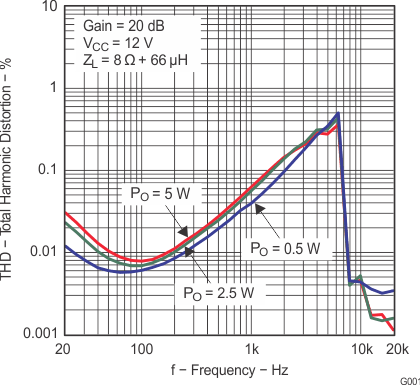Figure 1. Total Harmonic Distortion vs Frequency (BTL)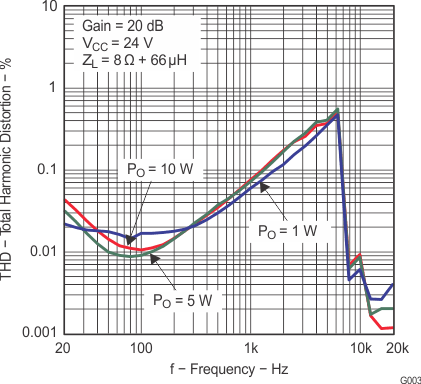Figure 3. Total Harmonic Distortion vs Frequency (BTL)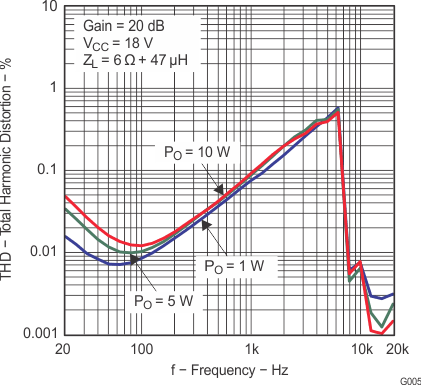Figure 5. Total Harmonic Distortion vs Frequency (BTL)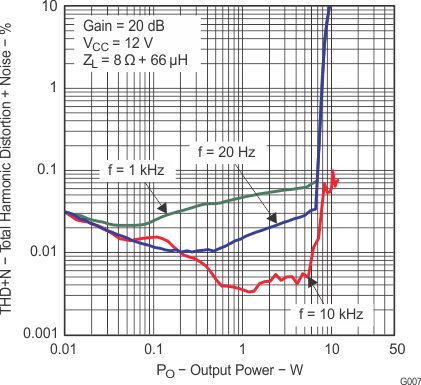Figure 7. Total Harmonic Distortion + Noise vs Output Power (BTL)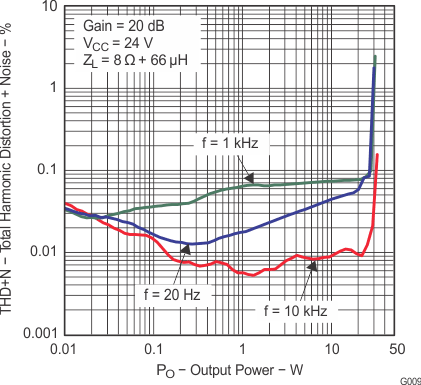Figure 9. Total Harmonic Distortion + Noise vs Output Power (BTL)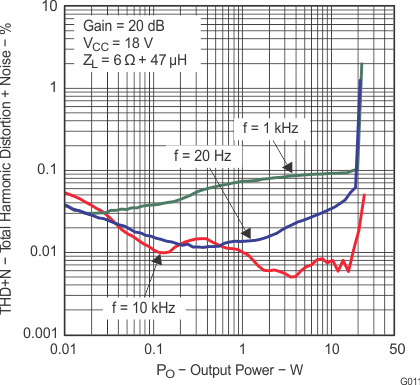Figure 11. Total Harmonic Distortion + Noise vs Output Power (BTL)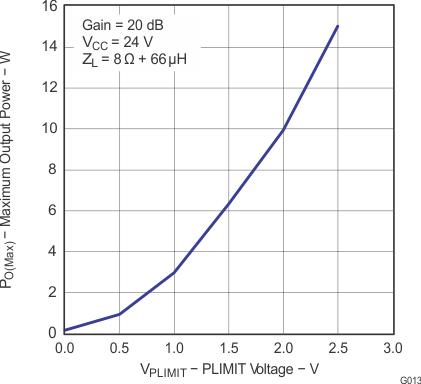SPACE
Figure 13. Maximum Output Power vs PLIMIT Voltage (BTL)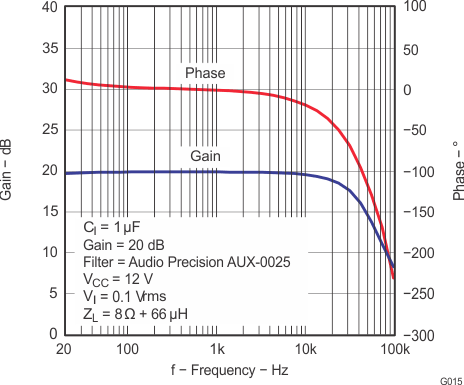SPACE
Figure 15. Gain and Phase vs Frequency (BTL)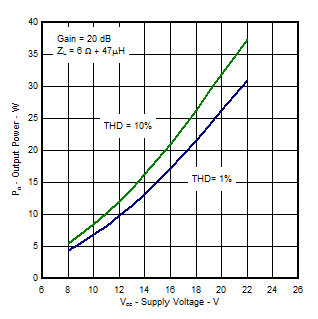The figure is measured with heatsink(1) on EVM(2)
Figure 17. Output Power vs Supply Voltage (BTL)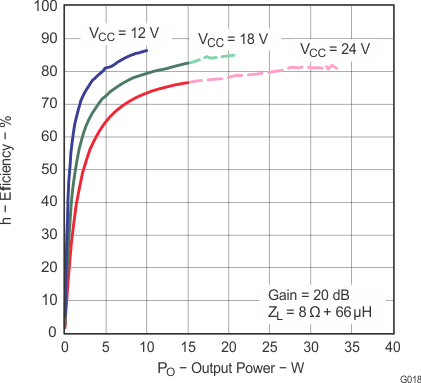Note: Dashed Lines represent thermally limited regions.
Figure 19. Efficiency vs Output Power (BTL)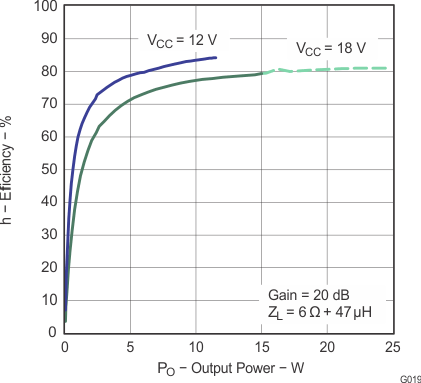Note: Dashed Lines represent thermally limited regions.
Figure 21. Efficiency vs Output Power (BTL)Figure 23. Efficiency vs Output Power (BTL)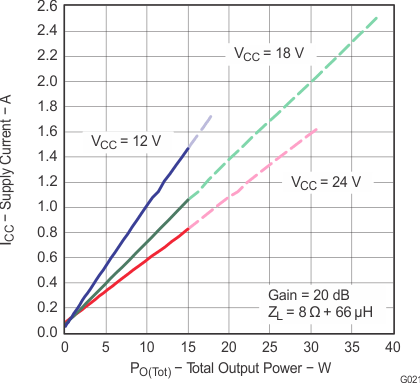Note: Dashed Lines represent thermally limited regions.
Figure 25. Supply Current vs Total Output Power (BTL)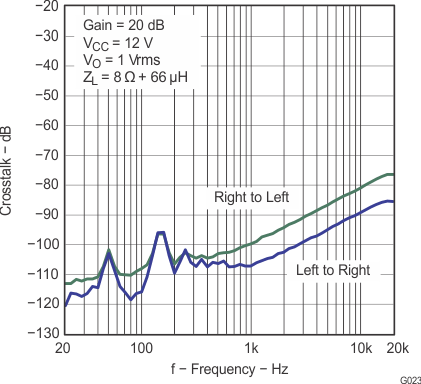SPACE
Figure 27. Crosstalk vs Frequency (BTL)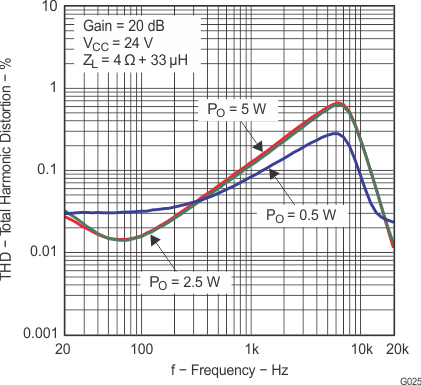Figure 29. Total Harmonic Distortion vs Frequency (PBTL)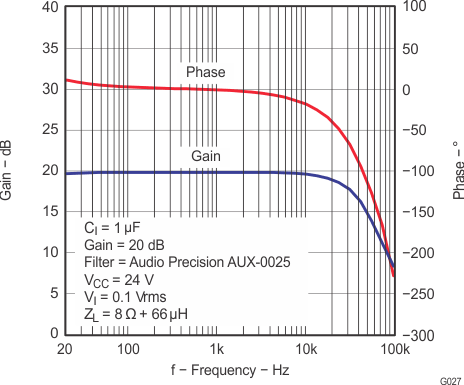Figure 31. Gain and Phase vs Frequency (PBTL)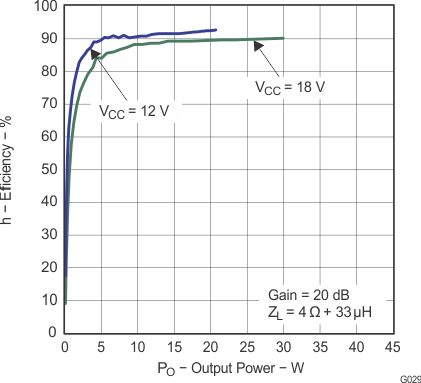SPACE
Figure 33. Efficiency vs Output Power (PBTL)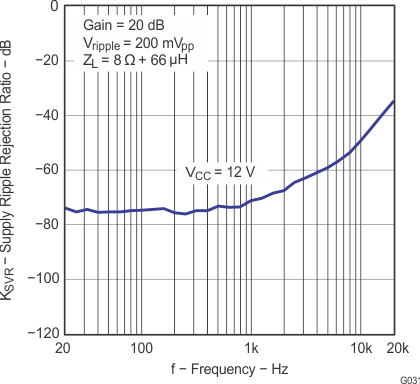Figure 35. Supply Ripple Rejection Ratio vs Frequency (PBTL)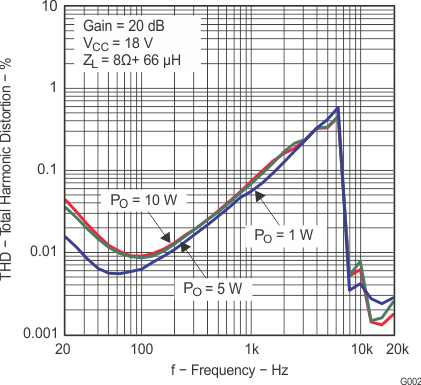Figure 2. Total Harmonic Distortion vs Frequency (BTL)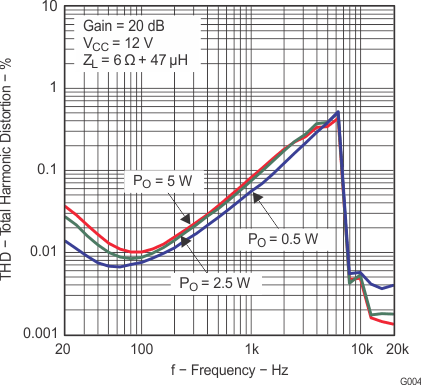Figure 4. Total Harmonic Distortion vs Frequency (BTL)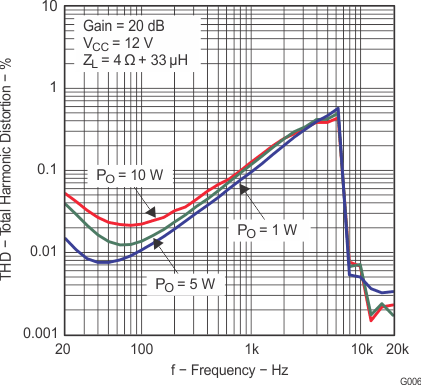Figure 6. Total Harmonic Distortion vs Frequency (BTL)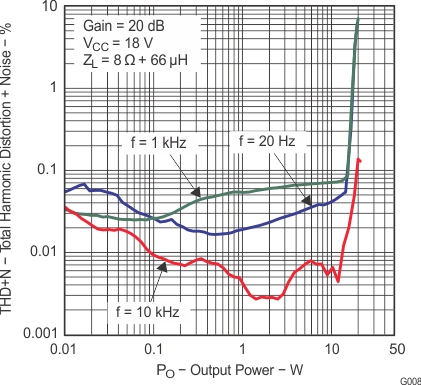Figure 8. Total Harmonic Distortion + Noise vs Output Power (BTL)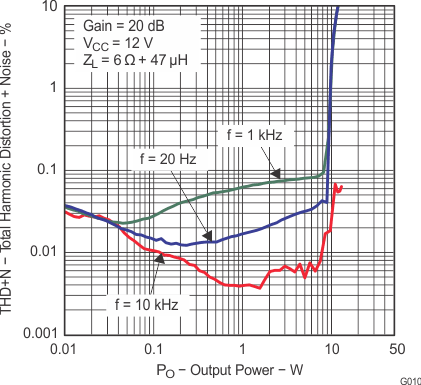Figure 10. Total Harmonic Distortion + Noise vs Output Power (BTL)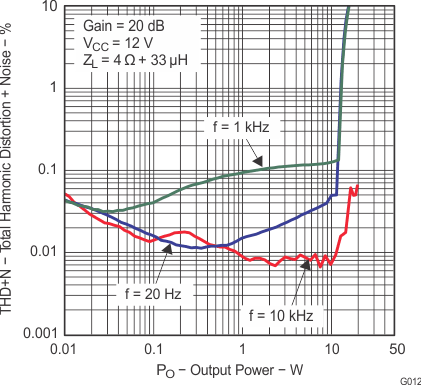Figure 12. Total Harmonic Distortion + Noise vs Output Power (BTL)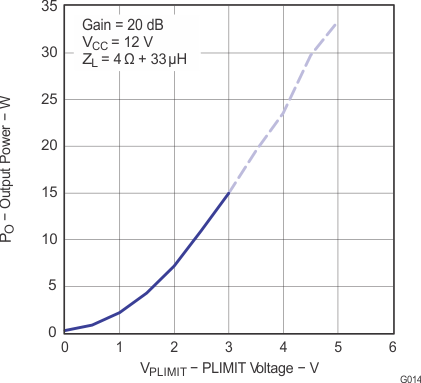Note: Dashed Lines represent thermally limited regions.
Figure 14. Output Power vs PLIMIT Voltage (BTL)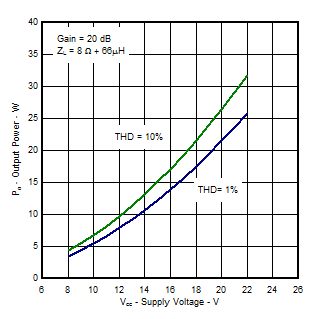The figure is measured with heatsink(1) on EVM(2)
Figure 16. Output Power vs Supply Voltage (BTL)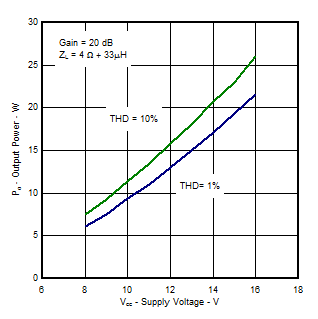The figure is measured with heatsink(1) on EVM(2)
Figure 18. Output Power vs Supply Voltage (BTL)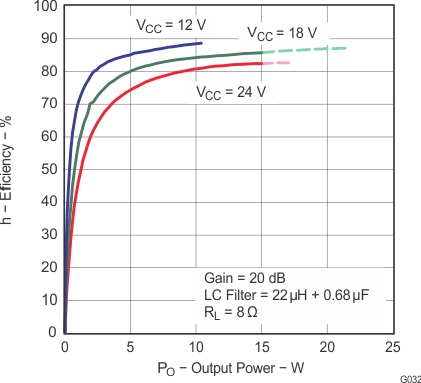SPACE
Figure 20. Efficiency vs Output Power (BTL With LC Filter)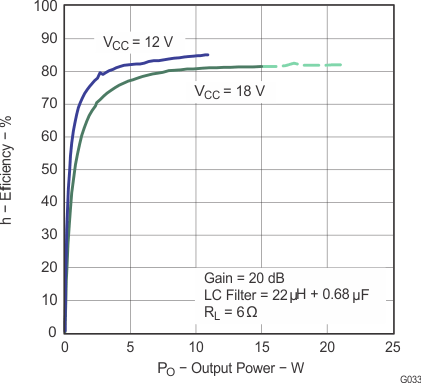Figure 22. Efficiency vs Output Power (BTL With LC Filter)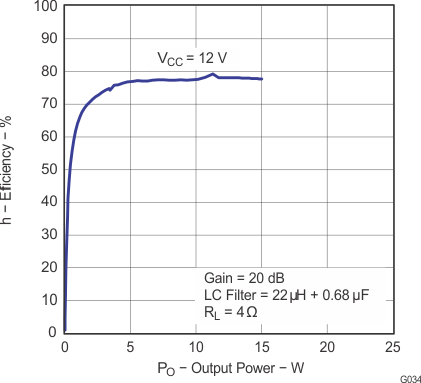SPACE
Figure 24. Efficiency vs Output Power (BTL With LC Filter)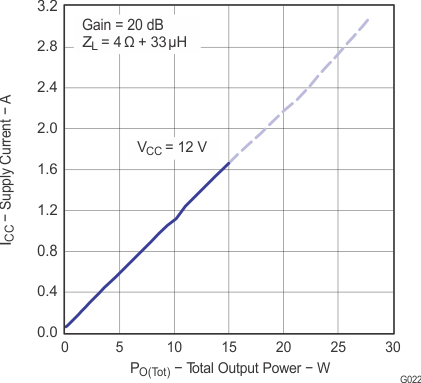Note: Dashed Lines represent thermally limited regions.
Figure 26. Supply Current vs Total Output Power (BTL)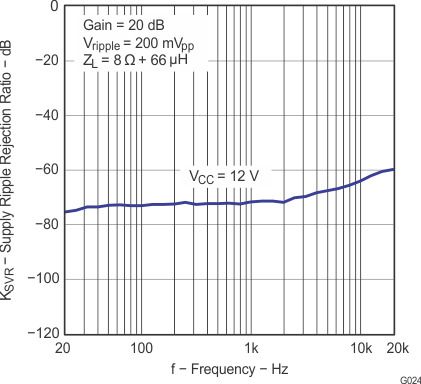Figure 28. Supply Ripple Rejection Ratio vs Frequency (BTL)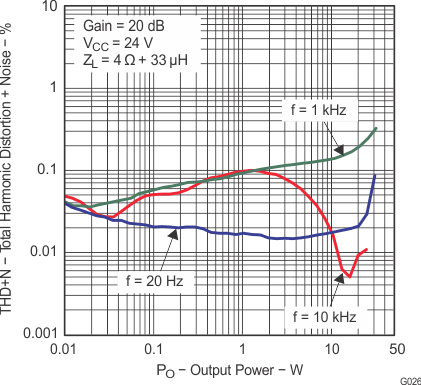Figure 30. Total Harmonic Distortion + Noise vs Output Power (PBTL)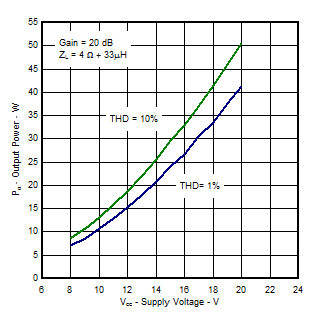The figure is measured with heatsink(1) on EVM(2)
Figure 32. Output Power vs Supply Voltage (PBTL)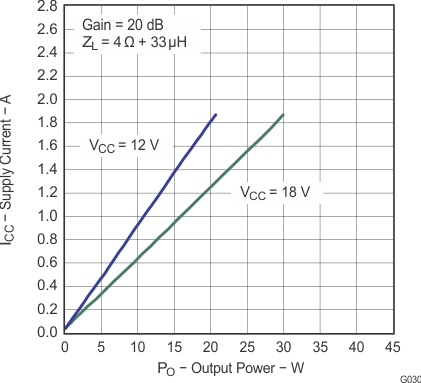Figure 34. Supply Current vs Output Power (PBTL)